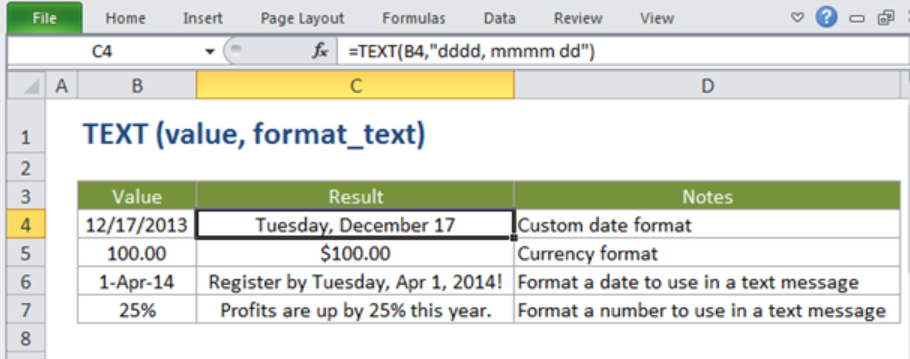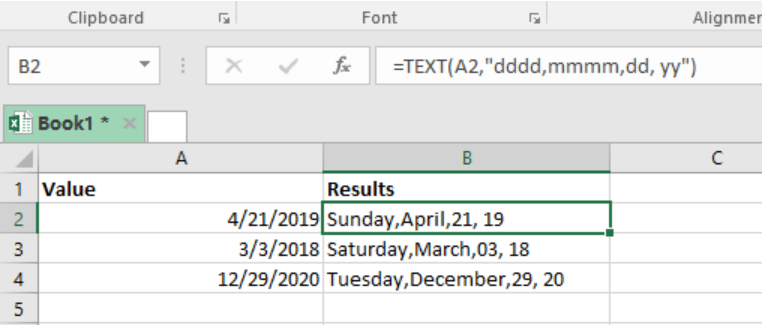Get instant live expert help with Excel or Google Sheets“My Excelchat expert helped me in less than 20 minutes, saving me what would have been 5 hours of work!”

#### Post your problem and you’ll get Expert help in seconds.

Your message must be at least 40 characters
Our professional Expert are available now. Your privacy is guaranteed.

# How to Use the Text Function in Excel

A time might come when we are required to convert a certain value into text. To do this, we will need to utilize the text function. In this post, we will look at how one can Format numbers as text.Figure 1: How to convert values to text_format

## General syntax of text function

`=TEXT(value, format_text)`

Where;

• Value- shows the number that we want to convert;
• Format_text- this one represents the number format that we wish to use in the operation.

## Explanation of text formula

The Excel text function is a fundamental function when we want to perform Excel text formatting to convert values into text in a given spreadsheet. In most cases, we use Excel TEXT Function when we want to convert a value into a text format in Excel. At the same time, one can use this text formula when we want to embed a formatted number into a text string. When this formula is applied to a given number, it will return a text value of the given numeric format.

The Excel text formula is designed to embed the numeric output of the formula or a given function and provide it in a given Excel text_format. Note that in order to use this formula and get the correct answer, then the text-format should be put into double quotation marks.

## Example of how to use text formatting

### Converting numeric dates to text formatFigure 2: Converting date values into text using text function

In this example, we want to convert the numeric date values into text. To do this, we need to prepare our Excel sheet table, with all the date values as shown in column A.

The next thing we need to do is to specify our formula, in column B. Given that we want to convert the date values in column A into text_format, we have to specify the exact format that we want the result to be displayed in. here, we have the day of the week, month, date and year. We should not forget that the Excel text formula utilizes double quotation marks to give us the desired results. Our formula will look like the one below;

`=TEXT(A2,"dddd,mmmm,dd, yy")`

Where;

• A2- represents the value,
• dddd- shows the day of the week;
• mmmm- shows the month of the year,
• yy- represents the year

After specifying the formula, we then need to press “Enter” to get the result in text_format.

To get the results of the other rows, all we need to do is copy down the text formula across the other cells in column B.

### Other uses of text function

We can use Excel text formula to convert values into other formats. We can convert values into currency format or even percentage. Using text function to convert values into percentage is a common practice when using spreadsheets.

## Conclusion

It is important to learn how to use the text formula. This is because this formula is fundamental when working with spreadsheets. Note that conversion of values into different formats is a common practice when working with Excel. Therefore, this skill will be a very useful one.

## Instant Connection to an Expert through our Excelchat Service

Most of the time, the problem you will need to solve will be more complex than a simple application of a formula or function. If you want to save hours of research and frustration, try our live Excelchat service! Our Excel Experts are available 24/7 to answer any Excel question you may have. We guarantee a connection within 30 seconds and a customized solution within 20 minutes.